Transcripted Summary

In this chapter, we're going to take a deeper look into data types in Java. Programming languages are either dynamically typed or statically typed.

Dynamically typed programming languages determine the data type of a variable at runtime — meaning while the program is running. Therefore, in these types of languages the programmer doesn't need to specify the data type of a variable.

Java is a statically typed programming language — which means, it expects its variables to be declared before they can be assigned values because, in statically typed languages the data type is checked at compile time.

### # Local Variable – Type Interference

While Java is a statically typed language, in version 10 of Java they added support for type inference — which means we can declare a variable as `var` and Java will infer the data type based on what is assigned to the variable.

Let's take a look at an example.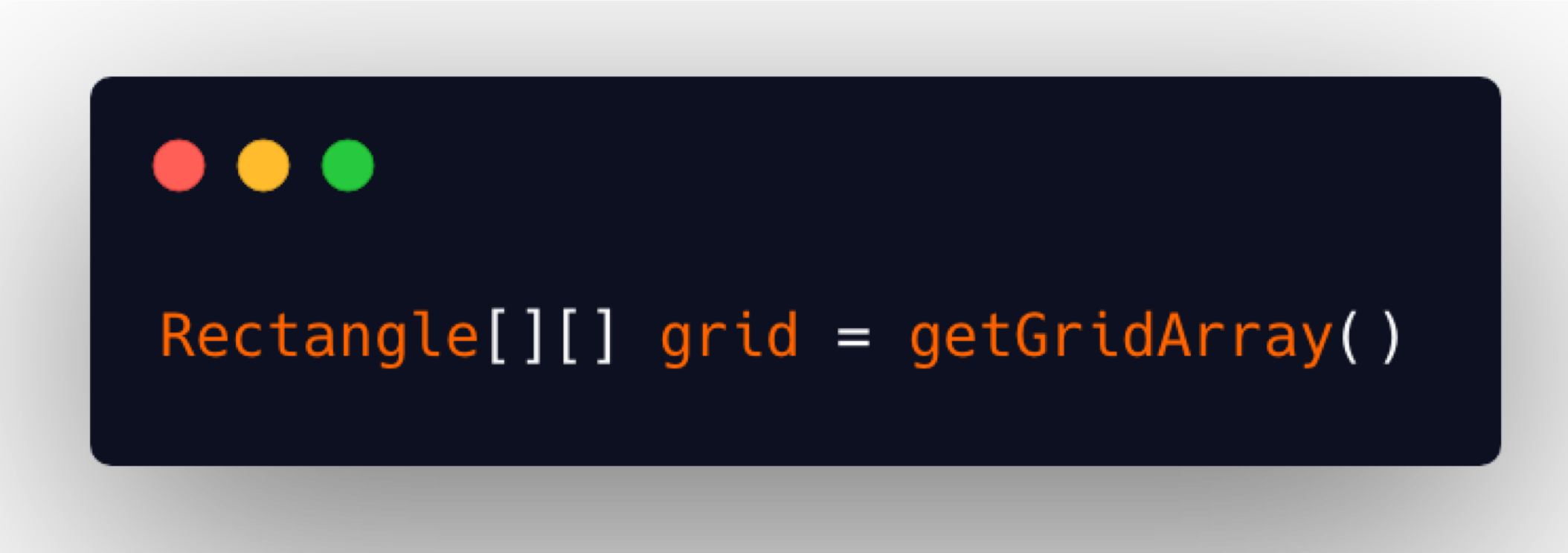Let's say we wanted to make a call to a method that returned a two-dimensional array of type `Rectangle` and we wanted to assign that to this variable `grid`. Before Java 10, we'd need to type out this data type, which is a bit annoying and cumbersome.

Now, with Java version 10 and later, we can simply use the reserved word `var` as the data type.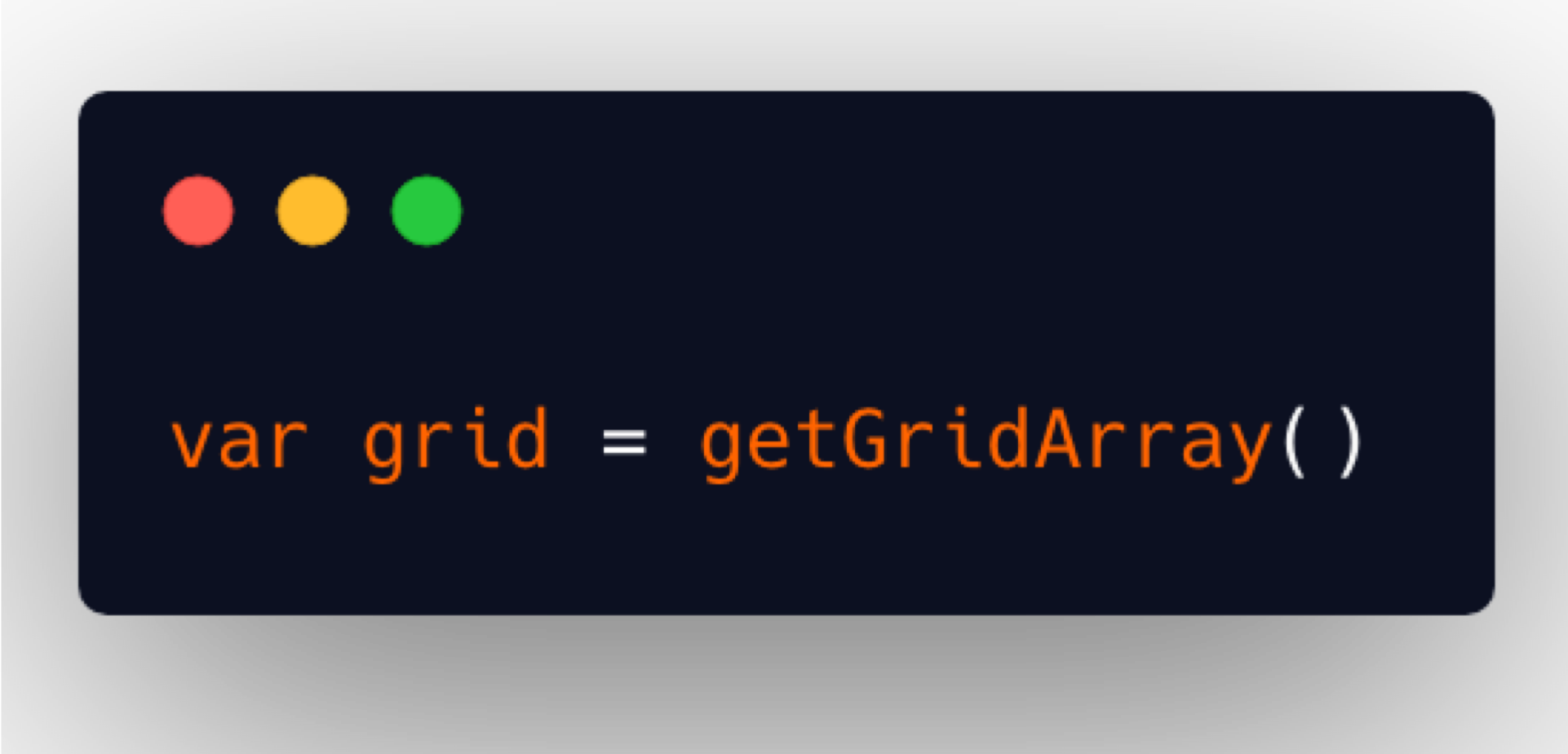With `var`, Java will infer that whatever is the data type of the value returned from the `getGridArray()` is what `grid` should be declared as. This is really nice, and it helps remove boilerplate code when working with more complex data types.

There are a few rules though.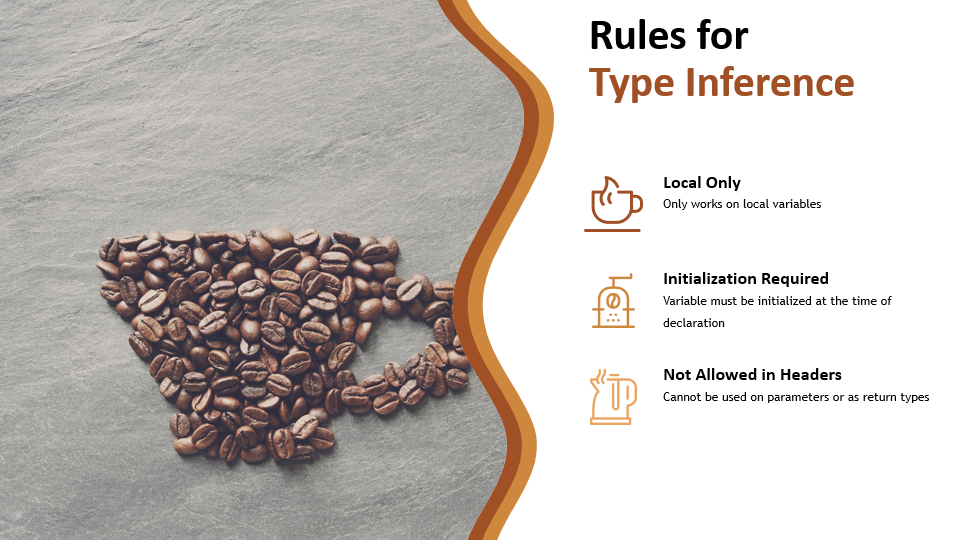Type inference variables are only allowed for local variables. You cannot use `var` for declaring a global variable.

You must initialize these variables at the time of declaration, otherwise, the compiler is unable to infer what the data type should actually be.

Type inference is not allowed in the headers of methods or constructors. You must still explicitly declare the data type of parameters as well as the return types of methods.

Let's talk some more about data types.

### # Primitive Data Types

One category of data types is primitive data types. These are the most basic data types and are provided by Java. There are 8 primitive data types. Throughout the course, we've already worked with half of them.

Here's the list of all 8 of them as well as their default values, their size, their type and an example.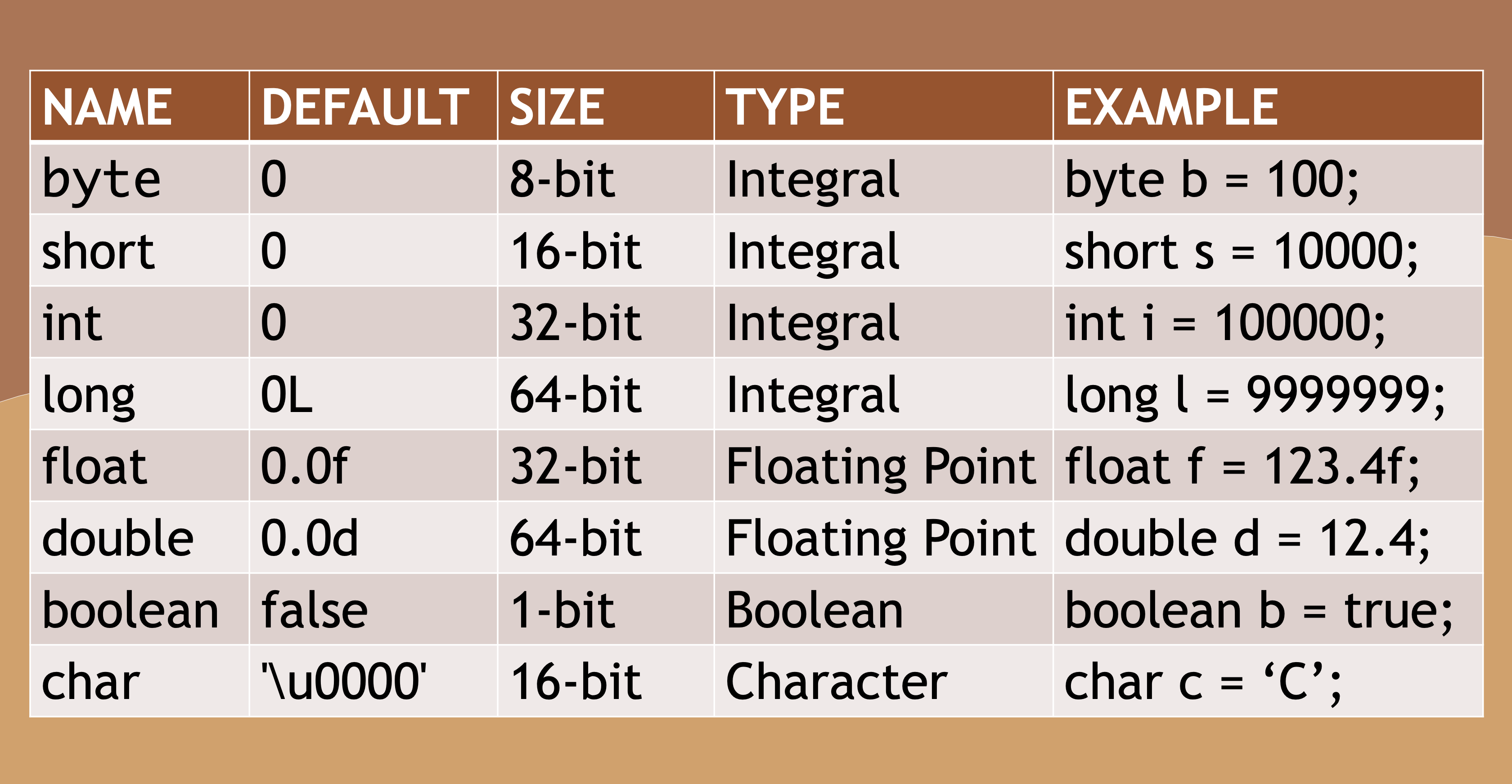Variables that are declared without an initial value are set to the default value — except for local variables, which you must initialize before using.

### # Wrapper Classes

All primitive data types can also be expressed as objects. These objects are known as wrapper classes.

Here we have two variables — `number1` and `number2`.

``````int number1 = 5;

Integer number2 = 5;
``````

Only `number1` has a data type of `int`, which is primitive.

And `number2` has a data type of `Integer`, which is a wrapper class for the primitive data type `int`. This makes `number2` an object, so that you can utilize convenience methods that are available in the `Integer` class.

Here's an example where we may want to convert decimal numbers into whole ones.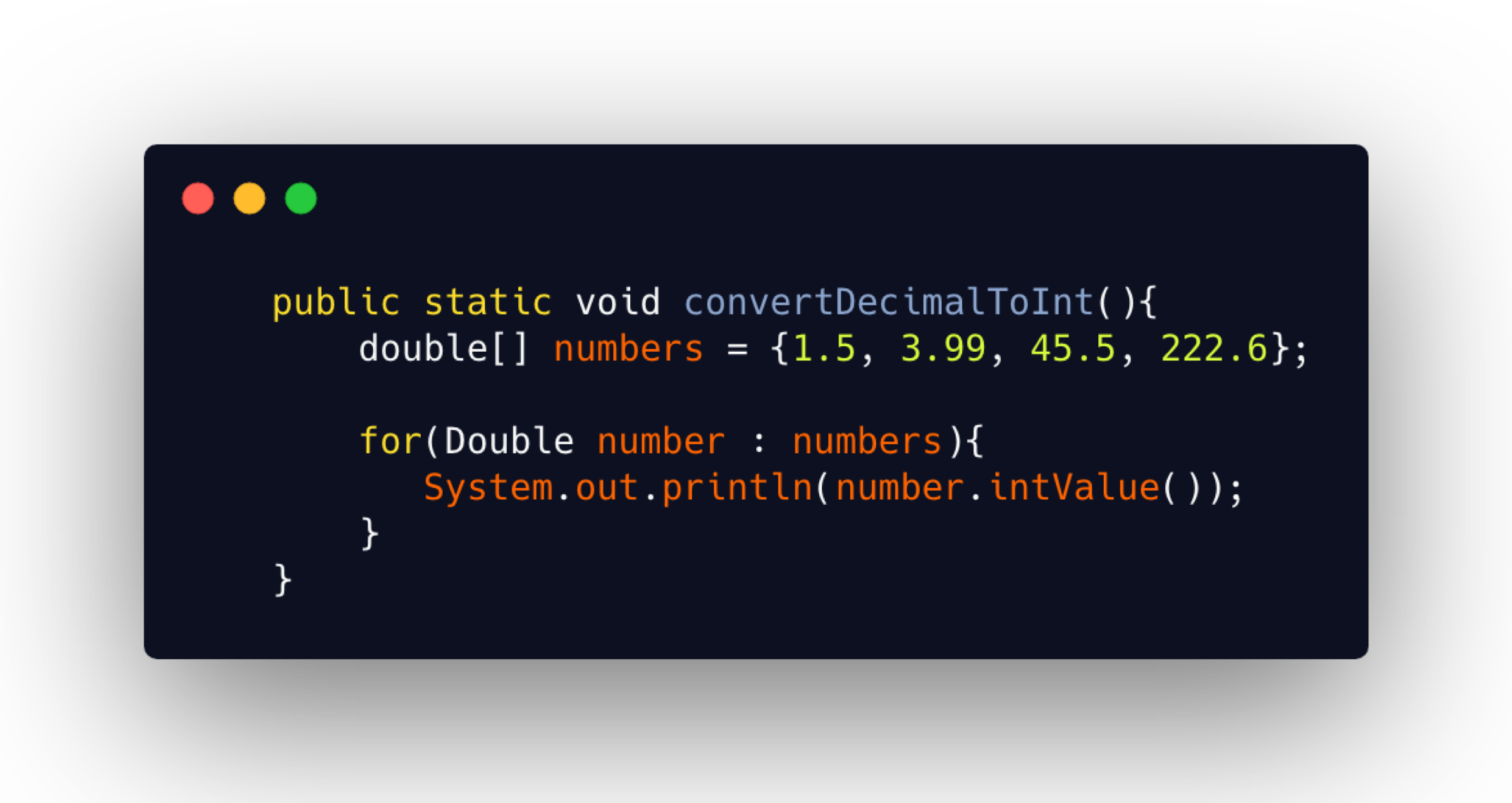We have an array of numbers declared as primitive type `double`. Yet, in the `for` loop, we have changed their type to its corresponding wrapper class denoted by the capital D in `Double`.

Now that this is an object, we can use the dot operator and make use of the methods, such as `intValue()` which will convert that decimal number into a whole number.

Here is the output from this run.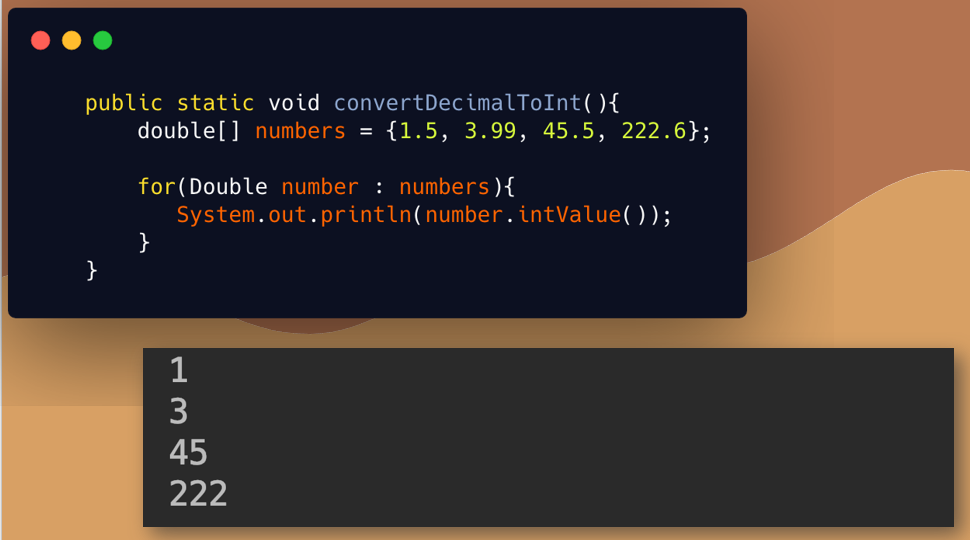In addition to methods to convert to other data types, there's also handy methods to do things like sum two numbers or get the min and max values allowed for the data type.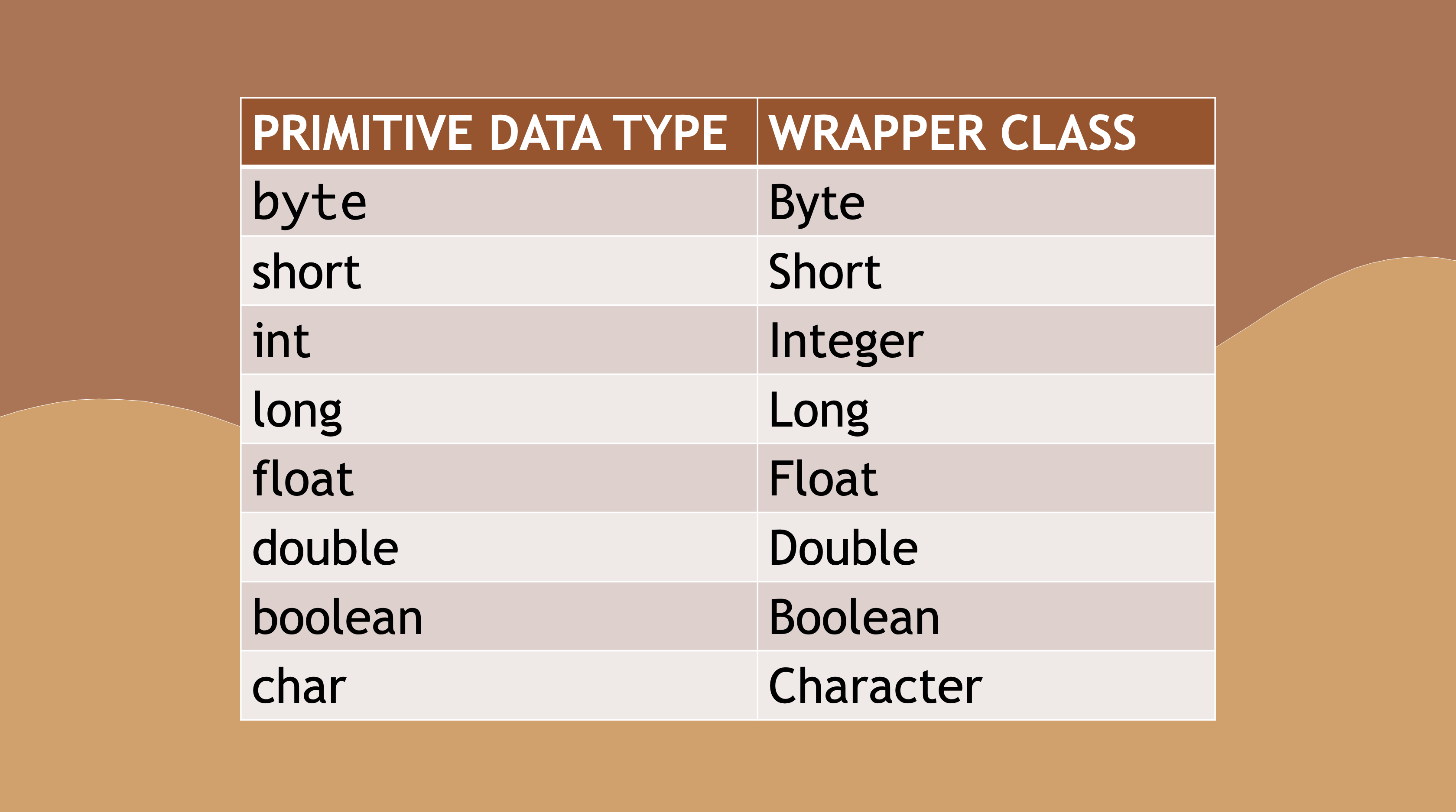These wrapper classes exist for all primitive data types.

Let's have a look at the Javadoc for one of these wrapper classes. This is the Integer class Javadoc.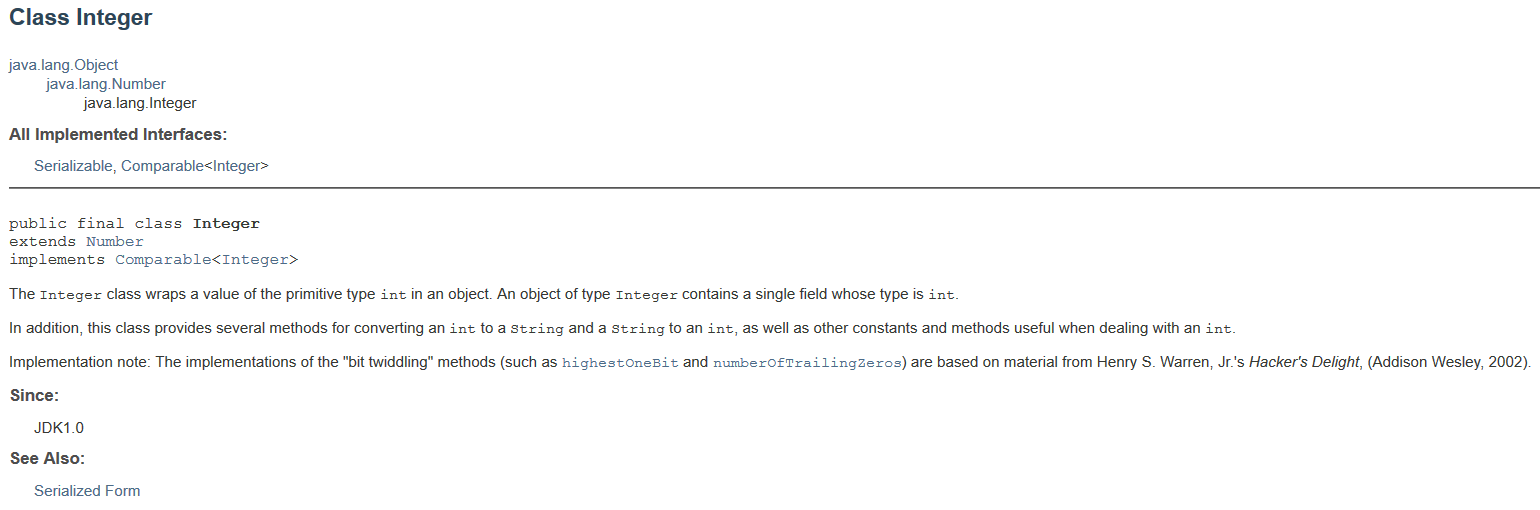Let's have a look at what's available here.

We notice that these are the fields.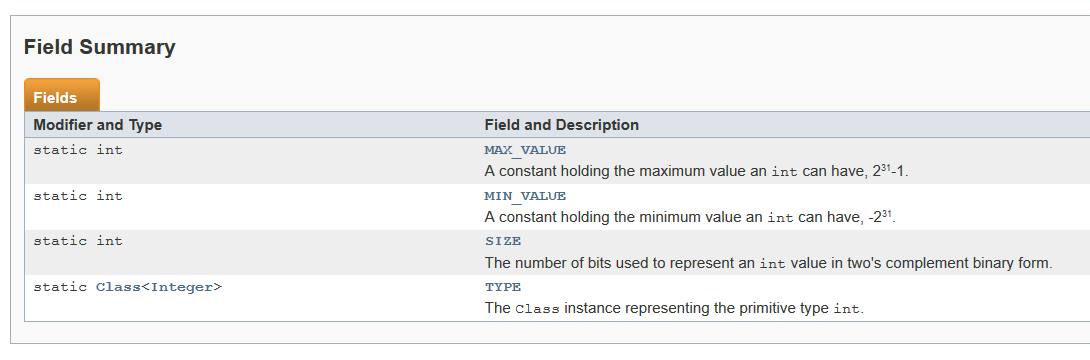All of them are `static`, meaning they can be used by just saying `Integer.` (with the dot operator) and you can get a bunch of these values like the `MAX_VALUE` value that's associated with an `Integer`, also the `MIN_VALUE`.

You can also see the methods.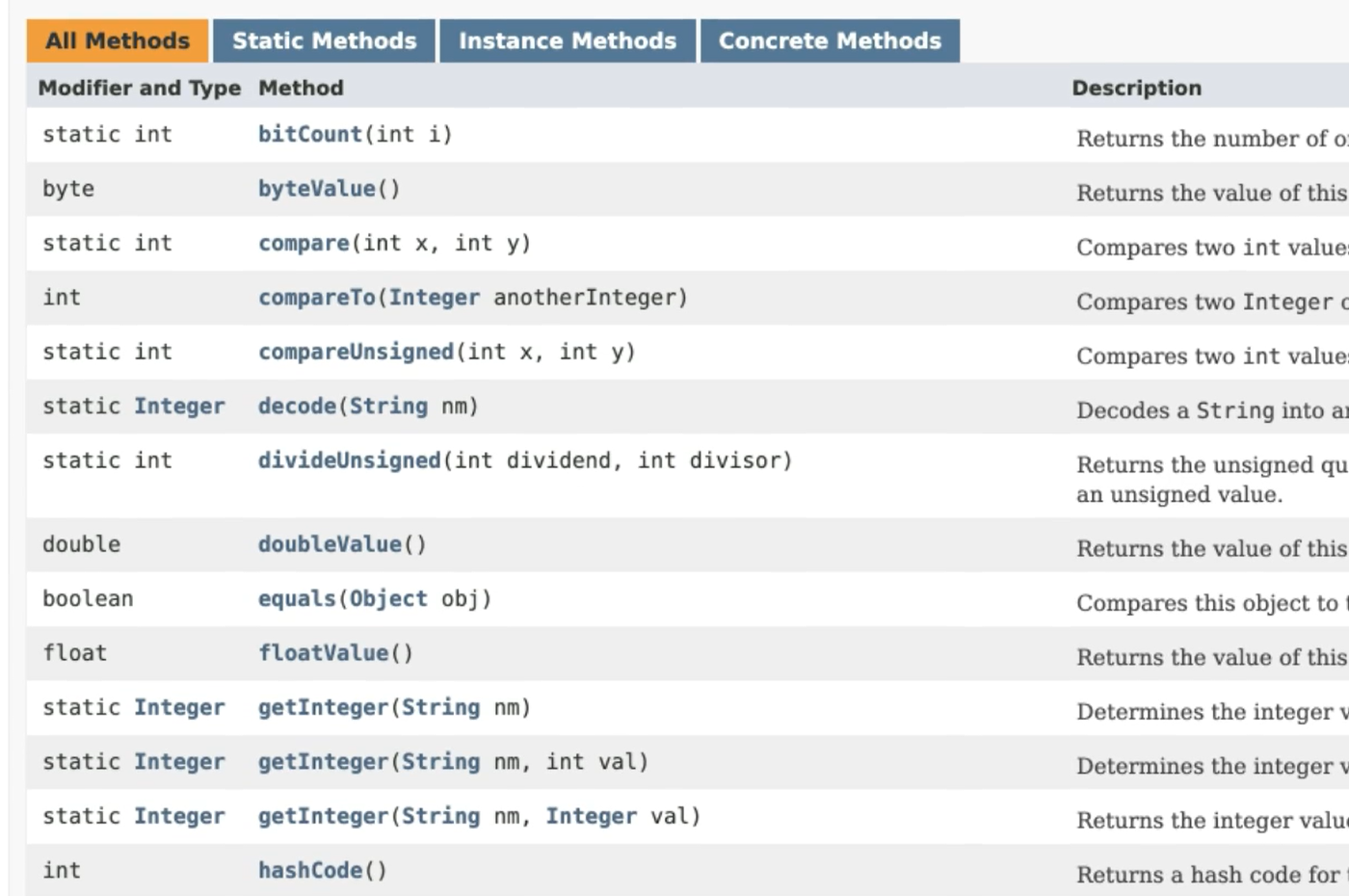Some of them are `static`, meaning they can be called with just the Integer class; `Integer.bitCount()` for example. And then some of them can be used from the instantiation of the object.

We have some useful ones here like `compare()` where you can pass in two numbers.

There’s `doubleValue()`, `floatValue()`, things like this to change it to a different data type in long value.

We have `max()` and `min()` where you can pass into numbers and get the max or the min of those two numbers.

All sorts of nice and useful things.

Also, this `valueOf()` is one that's pretty popular where you can pass in a String, which is just a number wrapped in quotation marks. Maybe you read this as input as a String, or from file or something that in its String value, but you can get the integer value of it.

Finally, you can turn an integer into a `String` by calling `toSring()`.

Quiz

The quiz for Chapter 8 can be found at the end of Section 8b